# How Bayes’ Theorem Coincides with Machine Learning

Just why is Bayes so naive?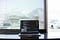Image Source

In this blog post, we’ll look at how to apply some of the theories for machine learning that are acquainted with Bayes’ theorem and foundational principles of Bayesian statistics. Classification problems are a natural implementation of Bayes’ theorem when you’re trying to predict a classification based on other data, which can be thought of as conditional probability. I will help you understand how to make a classification using the probabilities provided by Naive Bayes.

By believing that the features are autonomous of one another, Naive Bayes algorithms apply Bayes’ formula to several variables. The…

# Going Over Using K-Nearest Neighbors

A quick debriefing on a really cool supervised learning algorithm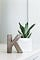Image Source

K-Nearest Neighbors, or KNN, is a supervised learning algorithm that can be applied on classification and regression problems. KNN is a distance-based classifier, which means it automatically implies that the closer two points are, the more identical they are. Euclidean distance, Minkowski distance, and Manhattan distance are all examples of different distance metrics. Each feature in KNN serves as a dimension. We can conveniently visualize this in a dataset of two columns by considering values for one column as X coordinates and the other as Y coordinates. Because this is…

# Accuracy and F1 Score — The Better Choices for Evaluating Model Success

Discussing the two most useful metrics that are used to describe a model’s efficiency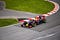Image Source

In my most recent blog post, I went over two of the easier and more common metrics used to explore model performance in machine learning, precision and recall. In this blog post, I will be discussing the two better choices for evaluating model performance — accuracy and F1 score — and going over how to evaluate them.

## Accuracy

The most logical metric is likely accuracy. Accuracy is helpful because it helps us to compute the amount of correct predictions a model makes because it includes true positives…

# Precision and Recall — What Are the Differences?

Precision and recall are two of the most fundamental evaluation metrics that we have at our hands.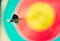Image Source

It’s imperative to compare your models to each other and pick the best fit models when performing tasks about classification. When you are estimating values in regression, it makes sense to speak about error as a deviation from the real values and how far apart the predictions were. But in classification, you are either correct or incorrect when classifying a binary variable. Consequently, we prefer to think of it in terms of how many false positives and false negatives a model has. In…

# Different Methods of Feature Selection in Machine Learning

How to automate the process of selecting features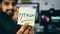Image Source

In data science, there are many different approaches to building features to model complicated relationships — although, this may sometimes be troublesome. But, you will learn about the various strategies you can use, in this blog post, to use only the features that are most important to your model!

## Defining Feature Selection

Feature selection is the approach by which you choose a subset of features specific to the design of models. This process comes with many advantages, with the most noticeable being the performance enhancement of a machine learning algorithm.

A second advantage includes decreasing…

# Derivatives are Confusing — So Here’s a 3 Minute Explanation

Hooray for calculus!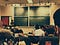Image Source

You have probably heard about the central principle of mathematical functions if you have studied linear regression. You can articulate this with the following example — assume that you have used the number of bathrooms in a house as a predictor and the house rental price as the target variable. The mathematical function of this example would be rental price = f(bathrooms), or more generically, y = f(x). Then, let’s assume that the price of the apartment is set in a very simplistic manner and the relation between the number of bathrooms and the rental price is…

# Regression, Big O Notation, and Gradient Descent — How Are They All Related?

A quick discussion on computational complexity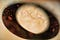Image Source

In this blog post, I will be exposing you to some of the complexity in computation in relation to OLS regression. You will read about this concept and see that this might not be the most powerful algorithm for estimating regression parameters while regression is being done with large datasets. This will lay the groundwork for an algorithm for optimization called gradient descent that will later be discussed.

In the case of simple linear regression, the OLS formula functions perfectly well because of a small number of computed operations. But, it gets computationally very…

# 4 Ways to Normalize and Scale Features for Regression Modeling

Improve your data to boost your regression results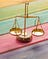Image Source

Normal features, or features that are as normally distributed as possible, will lead to better outcomes. This is what makes scaling and the normalization of features in regression modeling so significant. There are a number of ways to scale your features, and in this blog post, I am going to help you evaluate whether it is appropriate for a particular dataset or model to have normalization and/or standardization performed, while also having you consider the different approaches for standardization and normalization.

Sometimes there will be features that differ greatly in magnitude in…

# Dealing with Multicollinearity in Multiple Linear Regression

The biggest issue hindering quality results in regression modeling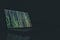Source

If you are familiar with data science, especially with regression modelling, then you are probably familiar with the concepts of covariance and correlation. This post will go over the issue of multicollinearity in multiple linear regression, go over how to create and interpret scatterplots and correlation matrices, and teach you how to identify if two or more predictors have collinearity.

## So… Why is Multicollinearity Bad?

The key purpose is to evaluate the relation between each predictor and the outcome variable when doing a regression analysis. The concept of a regression coefficient is that for every 1…

# Clarifying Regression Diagnostics for Linear Regression

How to test the required assumptions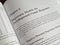Source

Regression diagnostics are a series of regression analysis techniques that test the validity of a model in a variety of ways. These techniques can include an examination of the underlying mathematical assumptions of the model, an overview of the model structure through the consideration of formulas with fewer, more, or unique explanatory variables, or an analysis of observation subsets, such as searching for those which are either badly represented by the data, like outliers, or that have a reasonably large effect on the predictions of the regression model. …## Acusio Bivona

Fitness, Sports, Data — And not necessarily in that order